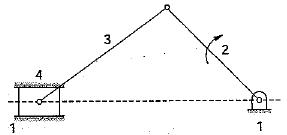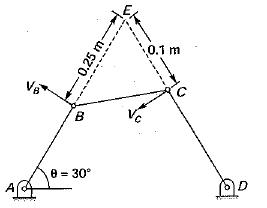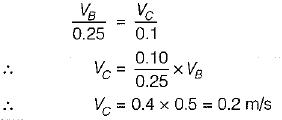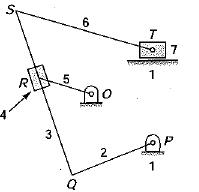Courses

# Test: Velocity & Acceleration Analysis - 1

## 10 Questions MCQ Test Topicwise Question Bank for Mechanical Engineering | Test: Velocity & Acceleration Analysis - 1

Description
This mock test of Test: Velocity & Acceleration Analysis - 1 for Mechanical Engineering helps you for every Mechanical Engineering entrance exam. This contains 10 Multiple Choice Questions for Mechanical Engineering Test: Velocity & Acceleration Analysis - 1 (mcq) to study with solutions a complete question bank. The solved questions answers in this Test: Velocity & Acceleration Analysis - 1 quiz give you a good mix of easy questions and tough questions. Mechanical Engineering students definitely take this Test: Velocity & Acceleration Analysis - 1 exercise for a better result in the exam. You can find other Test: Velocity & Acceleration Analysis - 1 extra questions, long questions & short questions for Mechanical Engineering on EduRev as well by searching above.
QUESTION: 1

Solution:

velocity = lω

= ω x AB

QUESTION: 2

### According to Kennedy's theorem, the instantaneous centers of three bodies having relative motion lie on a

Solution:

If three plane bodies have relative motion among themselves, their I-center must lie on a straight line, this is known as Kennedy’s theorem.

QUESTION: 3

### The instantaneous center of a slider moving in a curved surface lies

Solution:

In a sliding motion, the I-center lies at infinity in a direction perpendicular to the path of motion of the slider. The sliding motion is equivalent to a rotary motion of the links with the radius of curvature as infinity.

QUESTION: 4

The given figure shows a slider mechanism in which link 1 is fixed. The number of instantaneous centers would beSolution:
QUESTION: 5

A four bar mechanism ABCD is shown in the given figure. If the linear velocity ‘VB’ of the point ‘B’ is 0.5 m/s, then the linear velocity ‘Vc’ of point ‘C’ will beSolution:

E i s instantaneous center for the link A B and C D and angular velocity for the point B and C would be same about EQUESTION: 6

Examine the figure shown below wherein the numbers indicate the links:Which of the statements given below are correct?
1. I34 is at ∞, perpendicular to QS
2. I45 is at perpendicular to QS
3. I71 is at T
4. I45 is at R

Solution:
QUESTION: 7

A line drawn through an instantaneous center and perpendicular to the plane of motion is called instantaneous axis. The locus of this axis is known as

Solution:
QUESTION: 8

The instantaneous center of rotation of a circular disc rolling on a straight path is

Solution:
QUESTION: 9

The locus of instantaneous center of a moving body relative to a fixed body is known as the

Solution:

A centrode is the locus of the I-center of a plane body relative to another plane body for the range of motion specified or during a finite period of time.
Space centrode (or fixed centrode) is the locus of the I-center of the moving body relative to the fixed body.
Body centrode (or moving centrode) is the locus of the I-center of the fixed body relative to the movable body.

QUESTION: 10

The space centrode of a circular disc rolling on a straight path is

Solution: